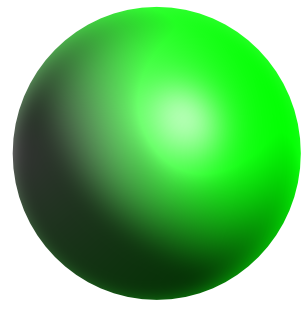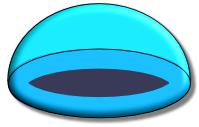Surface Area of a Sphere

## Surface Area of a SphereThe formula for the surface area of a sphere is:

A = 4pir^2

Note that the answer will be in square units eg cm2 or m2, as the answer is an area.

## Example 1

The diameter of a sphere is measured as 12cm. What, to 2 decimal places, is the surface area of the sphere?

 For a Sphere Area = 4pir^2 Substitute (r=frac(1)(2) xx 12) A = pi(6)^2 Divide both sides by 100 A = 113.0973

## Example 2

An experimental package is to be lowered into the sea to investigate global warming at the bottom of the ocean. Part of the experiment includes a hemisphere which is to be covered in a special coating. The hemisphere is solid, is of a constant thickness throughout and has no holes or apertures. The coating costs £4.50 per square centimetre.

The hemisphere has an external diameter of 40cm and has a constant thickness of 5mm. If the hemisphere has to be totally covered, what will the cost of the coating be?Total Surface Area = Surface area outside + surface area inside + rim

Radius of outside = 40 ÷ 2 = 20cm

Radius of inside = 20 - 5 = 15cm

 Area Sphereoutside Area = 4pir^2 Substitute A = 4pi(20)^2 = 5026.55 Area Sphereinside Area = 4pir^2 Substitute = 4pi(15)^2 = 2827.43 Area Rimouter Area = pir^2 Substitute = pi(20)^2 = 1256.64 Area Riminner Area = pir^2 Substitute = pi(15)^2 = 706.86

Total Surface Area

= 5026.55 + 2827.43 + (1256.64 - 706.86)

= 8403.76

Total Cost = 8403.76 x £4.50 = £37,816.92